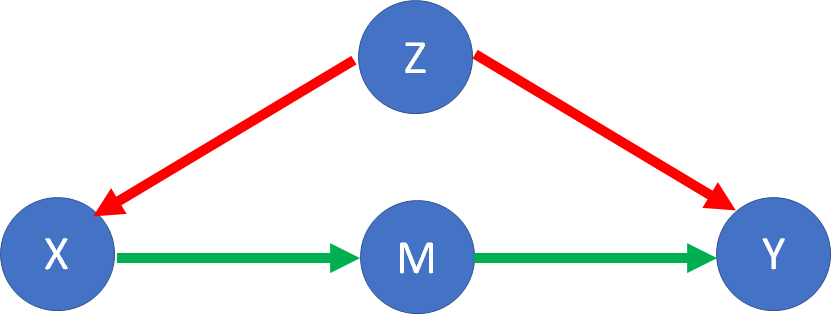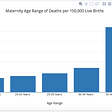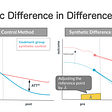# Regression and Causal Inference: Which Variables Should Be Added to The Model?

## Struggle and (Potential) Remedy

Oftentimes, the key purpose of data analysis is to provide insightful suggestions to some authority so that they can make changes to achieve a desirable outcome. For example, a supermarket owner may want to know whether offering a loyalty card to the customers will increase sales. This type of question is relevant in academia and in the public and the private sectors. Inherently, such questions are “causal” because by asking them we try to understand the causal effect of implementing a policy/program on an outcome. And, linear regression is the most common tool we use to answer such questions.

Regression, undoubtedly, is the most useful methodological tool that I have learned in my academic life. However, ever since I was introduced to multiple regression, the most puzzling question that I had was: other than the explanatory variable of my research interest, which other additional variables should I add to the model?

The applied statistics courses that I took initially, both online (in Coursera and EdX) and in-person, were focused on discussing how to estimate models using linear regression for “predictive” purposes. In these introductory /intermediate courses, for model building and variable selection, I learned to conduct partial F-tests. Simply put, we try two different models,

Full Model: Outcome = b0 + b1*Explanatory variable of Interest + b2*Additional Predictor + Error

Reduced Model: Outcome = b0 + b1*Explanatory variable of Interest + Error

Next, we do an F-test where,

the null hypothesis: b2=0 (models do not differ) and

the alternative hypothesis: b2 =/= 0 (models differ)

If the p-value of the F-statistic is less than 0.05, we reject the null hypothesis in favor of the alternative hypothesis and select the full model (which means we keep the “Additional Predictor” in the model). On the contrary, if the p-value is greater than 0.05, we fail to reject the null hypothesis and select the reduced model (which means we drop the “Additional Predictor” from the model).

Although useful, this test does not provide a framework to answer my question. Should I do partial F-tests with whatever variable I find in a dataset and make a decision based on the p-value? If that is the case, how many variables should I try?

The instructors suggested that the decision to add a variable should be made based on “theory”/ “subject matter knowledge”. And I kept wondering what does using theory/subject matter knowledge to select variables imply? Should I conduct a thorough literature review to identify all the predictors of the outcome, add them one by one to the model, and make a decision on whether they belong to the model based on the p-value of the partial F-tests?

Fortunately, last year, I took a course on “Causal Inference” and learned about the “Backdoor path criterion” which provides a framework to answer my question. Below, I will try to succinctly describe how to determine the set of additional variables (also known as control variables) that we need to add to a model for identifying and estimating the causal effect of interest.

# Step 1: Draw the Causal Graph

Let’s assume, we are interested in identifying and estimating the causal effect of a treatment (X) on an outcome (Y). If we are dealing with non-experimental data, at first, we have to figure out the possible paths through which statistical association can flow from X to Y. Basically, association from X to Y can flow in two types of paths: 1) causal paths 2) non-causal paths.

If X has any causal effect on Y, then the graph would be:

It is also possible that X causes M and then M causes Y. In this case, M is a “descendant” of treatment X and is known as a “Mediator”.Figure 2*: An indirect Causal Path from X to Y. Here, M is a mediator.

Now, regardless of X having a direct/indirect causal effect on Y, an association can flow from X to Y through non-causal paths, such as backdoor paths.

For example, it is possible that both X and Y are caused by a common factor Z. Such a common factor is called a “Confounder”. In the graph shown below, an association can flow from X to Y through the backdoor path X ← Z → Y.Figure 3*: If not controlled for, Z confounds the causal relationship between X and Y as X ← Z →Y backdoor path is open. The resulting bias is known as confounding bias/omitted variable bias.

Backdoor Path: Any path from X to Y with an arrow pointing towards X is a backdoor path.

Let’s pretend that we did a thorough literature review and/or applied our subject matter knowledge and came up with the following complete graph showing all the causal and non-causal paths from X to Y:

Next, we identify all the backdoors (marked in red) through which unwanted information can flow from X to Y:

X ← Z →Y

X ← Z2 ← Z1 → Z →Y

X ← Z ← Z3 → Z4 →Y

Is that all?

No! Actually, there is another backdoor path from X to Y:

X ← Z2 ← Z1 → Z ← Z3 → Z4 → Y

In this path, Z is not a confounder, rather a collider. A collider is a variable that is a common effect of two other variables; here, Z is the common effect of Z1 and Z3.

Interestingly, when a collider is not controlled for, the backdoor path remains blocked, i.e., no unwanted information flows through the path; but, if controlled for, the backdoor path becomes open.

# Step 2: Satisfy the Backdoor Path Criterion

To identify the causal effect of X on Y, the backdoor path criterion says, we need to control for a set of variables which:

1. contains no descendent of X,

2. blocks every backdoor path from X to T.

So, now, we have finally found a framework to decide on which additional variables should be added to the model!

Let’s try to determine the set of additional variables (control variables):

1. As M is a descendent of X, M cannot be part of the set.
2. To block the backdoor path X ← Z →Y, we need to add Z to the set of control variables.
3. To block the backdoor path X ← Z1 ← Z2 → Z →Y, we can add any one, two, or all of Z1, Z2, and Z to the set.
4. To block the backdoor path X ← Z ← Z3 → Z4 →Y, we can add any one, two, or all of Z, Z3, and Z4 to the set.

Looking at points 2, 3, and 4 mentioned above, initially, we might assume that controlling for only Z is sufficient to block all three backdoor paths. However, as Z is a collider in the backdoor path X ← Z2 ← Z1 → Z ← Z3 → Z4 → Y, controlling for Z opens it.

Then, what is the solution?

We must control for Z as it is the only way to block the backdoor path X ← Z →Y. And, to block the newly opened backdoor path X ← Z2 ← Z1 → Z ← Z3 → Z4 → Y, we can control for either Z2, or Z1, or Z3, or Z4.

So, the minimum sufficient adjustment set (set of variables to control for by adding them to the model) to satisfy the backdoor path criterion can be S= {Z, Z1}, or S={Z, Z2}, or S = {Z, Z3}, or S = {Z, Z4}.

To estimate the causal effect of X on Y, we can fit any of the following four models:

Y = b0 + b1*X + b2*Z + b3*Z1 + error

Y = b0 + b1*X + b2*Z + b3*Z2 + error

Y = b0 + b1*X + b2*Z + b3*Z3 + error

Y = b0 + b1*X + b2*Z + b3*Z4 + error

Obviously, if we have data on all of Z, Z1, Z2, Z3, and Z4, we can add all of them to the model as well:

Y= b0 + b1*X + b2*Z + b3*Z1 +b4*Z2 + b5*Z3 + b6*Z4+error

However, while working with non-experimental data (mostly from surveys), often, we do not have access to all the variables. So, the key question is: do we have data on the minimum number of variables needed to satisfy the backdoor path criterion? If yes, then we can identify and estimate the causal effect of interest. Otherwise, we cannot depend on just a multiple regression like the ones shown above and have to come up with an alternative strategy (e.g., difference-in-difference, instrumental variable, regression discontinuity, etc.) to identify and estimate the causal effect.

If you would like to read some more beginner-level articles on causal inference, read some of my previous posts:

Reference

Pearl, J., Glymour, M., & Jewell, N. P. (2016). Causal inference in statistics: A primer. John Wiley & Sons. http://bayes.cs.ucla.edu/PRIMER/

--

--

--

## More from Vivekananda Das

Sharing ideas on Cause-and-Effect, Data Analysis in R, Stat Literacy, and Happiness | Ph.D. student @UW-Madison | More: https://vivekanandadas.com

Love podcasts or audiobooks? Learn on the go with our new app.

## Interrogating Full GPT-2## Demystifying Bubble Sort## Python Data Visualization — Comparing 5 Tools## Udacity Data Visualization Nanodegree: Capstone Project## Step by Step Tutorial — Create a bar chart race animation## Did AI select you as a candidate for a promotional offer?## Vivekananda Das

Sharing ideas on Cause-and-Effect, Data Analysis in R, Stat Literacy, and Happiness | Ph.D. student @UW-Madison | More: https://vivekanandadas.com

## Causal inference using Synthetic Difference in Differences with Python## CAUSAL INFERENCE COURSES (CORRELATION DOES NOT IMPLY CAUSATION !)## Hyperparameter tuning for hyperaccurate XGBoost model## Causation, Not Correlation — The Nature of Causal Inference